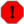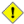## Name

= — Returns `TRUE` if A's bounding box is the same as B's.

## Synopsis

`boolean =(` geometry A , geometry B `)`;

## Description

The `=` operator returns `TRUE` if the bounding box of geometry A is the same as the bounding box of geometry B. PostgreSQL uses the =, <, and > operators defined for geometries to perform internal orderings and comparison of geometries (ie. in a GROUP BY or ORDER BY clause).This is cause for a lot of confusion. When you compare geometryA = geometryB it will return true even when the geometries are clearly different IF their bounding boxes are the same. To check for true equality use ST_OrderingEquals or ST_EqualsThis operand will NOT make use of any indexes that may be available on the geometries.

## Examples

```SELECT 'LINESTRING(0 0, 0 1, 1 0)'::geometry = 'LINESTRING(1 1, 0 0)'::geometry;
?column?
----------
t
(1 row)

SELECT ST_AsText(column1)
FROM ( VALUES
('LINESTRING(0 0, 1 1)'::geometry),
('LINESTRING(1 1, 0 0)'::geometry)) AS foo;
st_astext
---------------------
LINESTRING(0 0,1 1)
LINESTRING(1 1,0 0)
(2 rows)

-- Note: the GROUP BY uses the "=" to compare for geometry equivalency.
SELECT ST_AsText(column1)
FROM ( VALUES
('LINESTRING(0 0, 1 1)'::geometry),
('LINESTRING(1 1, 0 0)'::geometry)) AS foo
GROUP BY column1;
st_astext
---------------------
LINESTRING(0 0,1 1)
(1 row)```